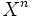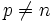# Cellular filtration

(Redirected from Cellular space)

## Definition

A filtration of a topological space (say$X^n$ the filtration for$X$) is said to be a cellular filtration if it satisfes the following conditions:

•$H_p(X_n,X_{n-1}) = 0$for all$p \ne n$
• For any singular simplex, we can find a$n$ such that the image of the simplex sits inside$X^n$

A topological space equipped with the additional structure of a cellular filtration is termed a cellular space.

## Relation with other structures

### Stronger structures

• CW complex: The structure of a CW complex on a topological space automatically gives it a cellular filtration